#DC学院学习笔记（二十一）：用特征选择方法优化模型（二）

0
0
01. 云栖社区>
2. 博客>
3. 正文

## DC学院学习笔记（二十一）：用特征选择方法优化模型（二）

kissjz 2018-02-19 15:24:54 浏览1544

# 随机森林特征选择法 —— Gini Importance

Gini index：衡量决策树每一棵树上的节点上面所存在的数据的纯净度的一个指标。这个值越小，纯净度越高。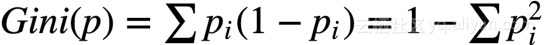$p_{i}$是节点内各个特征所占的概率。

OK,原理不再多说了。

## 更多请看我之前写的博客：

Gini decrease：每个节点的Gini index - 子节点的Gini index之和（这里的和是加权和）。

Gini importance：将所有树上相同特征节点的Gini decrease加权的和。

1、这种方法存在偏向，对具有更多取值的特征会更有利
2、对于存在关联的多个特征，其中任意一个都可以作为指示器（优秀的特征），并且一旦某个特征被选择之后，其他特征的重要度就会急剧下降，因为不纯度已经被选中的那个特征降下来了，其他的特征就很难再降低那么多不纯度了，这样一来，只有先被选中的那个特征重要度很高，其他的关联特征重要度往往较低。在理解数据时，这就会造成误解，导致错误的认为先被选中的特征是很重要的，而其余的特征是不重要的，但实际上这些特征对响应变量的作用确实非常接近的（这跟Lasso是很像的）。

Python实现

import pandas
import numpy as np
from sklearn import ensemble
from sklearn.preprocessing import LabelEncoder

iris.columns=['SepalLengthCm','SepalWidthCm','PetalLengthCm','PetalWidthCm','Species']

le = LabelEncoder()
le.fit(iris['Species'])
rf = ensemble.RandomForestClassifier()
features = ['PetalLengthCm','PetalWidthCm','SepalLengthCm','SepalWidthCm']
y = np.array(le.transform(iris['Species']))
X = np.array(iris[features])

#Gini importance
rf.fit(X,y)

print(rf.feature_importances_)
[ 0.34870355  0.52788187  0.10220203  0.02121255]



# 随机森林特征选择法 —— Mean Decrease Accuracy

1.训练出一个随机森林模型，在测试集检验得到accuracy0;
2.随机重排(permutation)测试集某特征xi，检验得到accuracyi;
3.(accuracy0 - accuracyi)/accuracy0,即为特征xi的重要性。

Python实现

from sklearn.metrics import accuracy_score
from sklearn.model_selection import ShuffleSplit

rs = ShuffleSplit(n_splits=10,test_size=0.1)
scores = np.zeros((10,4))
count = 0
for train_idx, test_idx in rs.split(X):
X_train , X_test = X[train_idx] , X[test_idx]
y_train , y_test = y[train_idx] , y[test_idx]
r = rf.fit(X_train,y_train)
acc = accuracy_score(y_test,rf.predict(X_test))
for i in range(len(features)):
X_t = X_test.copy()
np.random.shuffle(X_t[:, i])
shuff_acc = accuracy_score(y_test,rf.predict(X_t))
scores[count,i] = ((acc-shuff_acc)/acc)
count += 1
print(np.mean(scores,axis=0))
[ 0.24150183  0.35630037  0.00666667  0.        ]



# 线性回归特征选择：L1正则化Lasso

1、约束参数，降低模型复杂度。
2、规则项的使用还可以约束我们的模型的特性。这样就可以将人对这个模型的先验知识融入到模型的学习当中，强行地让学习到的模型具有人想要的特性，例如稀疏、低秩、平滑等等。

L1范数：向量中各个元素绝对值之和，也有个美称叫“稀疏规则算子”（Lasso regularization）。

L1范数的作用：由于L1范数的天然性质，对L1优化的解是一个稀疏解，因此L1范数也被叫做稀疏规则算子。通过L1可以实现特征的稀疏，去掉一些没有信息的特征，例如在对用户的电影爱好做分类的时候，用户有100个特征，可能只有十几个特征是对分类有用的，大部分特征如身高体重等可能都是无用的，利用L1范数就可以过滤掉。

L1正则化Lasso

• 普通线性回归：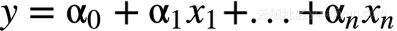• 普通线性回归目标函数：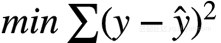• Lasso目标函数：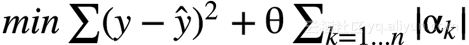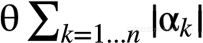L1正则化python实现

import pandas
import numpy as np
from sklearn import linear_model
from sklearn.preprocessing import LabelEncoder

le = LabelEncoder()
le.fit(iris['Species'])
lm = linear_model.Lasso(0.02)
features = ['PetalLengthCm','PetalWidthCm','SepalLengthCm','SepalWidthCm']
y = np.array(le.transform(iris['Species']))
X = np.array(iris[features])

lm.fit(X,y)
print(lm.coef_)
[ 0.28777819  0.35160589 -0.         -0.        ]


kissjz
+ 关注